# Variations in HYP, CNTR and GenMP

This page describes the introduction of variations in the HYP, HYP_OR, CNTR and GENMP reasoners.

# Context

The reasoners implementing the inference rules

HYP

,

HYP_OR

,

CNTR

and

GENMP_*

look for exact matches of a predicate. But, quite often, a similar predicate that does not match exactly (e.g., because it has been normalized) would suffice. There is also the possibility that a variation which is equivalent to the predicate would be present. For instance, "¬ a = b" is equivalent to "¬ b = a". Finally, the reasoners shall also consider variations which are a sufficient condition for the rule. For instance, if the goal is "a ≤ b", then a hypothesis "a < b" is a sufficient condition to discharge the goal with

HYP

.

# Objective

The point of this enhancement is therefore to increase the proof power of the considered reasoners by having them consider variations of the sought predicate.

# Conventions

The variations in the Inference Rules page have been generated such that the following facts hold:

•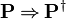$\textbf{P} \limp \textbf{P}^{\dagger}$
•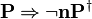$\textbf{P} \limp \lnot \textbf{nP}^{\dagger}$

Moreover, we consider only variations that are in normal form (that is invariant under rewriting by the auto-rewriter of the sequent prover). This is why the table does not contain predicates such as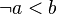$\lnot a < b$, but contain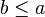$b\le a$ instead.

# Implementation

This section explains how the generation of variation of the sought predicate has been implemented.

## Variation Implementation

In order to simplify and optimize the generation of variation of the sought predicate, 4 functions (common to HYP, HYP_OR, CNTR and GenMP reasoners) have been implemented. Each function takes as input a predicate and returns a list of predicates generated by applying their corresponding rule.

These functions have been defined as follows:

StongerPositive(P) =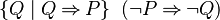$\{Q \mid Q \limp P\} \;\; (\lnot P \limp \lnot Q)$
WeakerPositive(P) =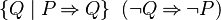$\{Q \mid P \limp Q\} \;\; (\lnot Q \limp \lnot P )$
StongerNegative(P) =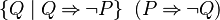$\{Q \mid Q \limp \lnot P\} \;\; (P \limp \lnot Q)$
WeakerNegative(P) =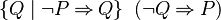$\{Q \mid \lnot P \limp Q\} \;\; (\lnot Q \limp P )$

A rule can be written in 2 implications, one for each sign of the predicate P.

Some optimisations, to reduce the number of implication to implement, have been implemented. Indeed, only the implications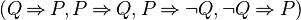$(Q \limp P, P \limp Q, P \limp \lnot Q, \lnot Q \limp P)$ have been implemented because they cover all cases. For instance, if the function StongerPositive takes a negative predicate as an input, the function returns a list of predicates stronger (e.g which infer) than the input. But it's also performed by the function StongerNegative. So this function applies the predicate negation and call to StongerNegative function.

Note: These functions assume the operators such as '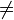$\neq$' have been removed by the normalization. Moreover, as explained in the convention part, the list of predicates returned contains only predicates in a normal form.

## Reasoners Implementation

### HYP, HYP_OR

The HYP reasoner calls to StongerPositive function in order to generate a list of predicates that infer the goal. Thus, the sequent is discharged if and only if at least one hypothesis is contained into this list.

The HYP_OR reasoner proceeds the same, for each disjunct a list of stronger predicates is generated and then the sequent is dicharged if at least one hypothesis is contained into these lists.

### CNTR

Checks if the predicate, that we would contradict, is not null and it is contained into the hypotheses.

To generate the predicates contradicting the given hypothesis, a list of predicates inferring the predicate is generated if the given hypothesis is a positive predicate. Else, the reasoner splits a possible conjunctive hypothesis, into its conjuncts and computes for each conjunct a list of predicates inferring the negation of the predicate (or conjunct). Finally, the sequent is dicharged if at least one predicate computed is contained into the hypotheses. If so, all hypotheses needed to achieve it are stored in the list named neededHyps.

### GEN_MP

For each predicate extracted (unless predicates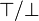$\btrue / \bfalse$) from the hypotheses the reasoner computes:

• a list of predicates which could be remplaced by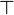$\btrue$ and which are weaker than the hypothesis.
• a list of predicates which could be remplaced by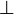$\bfalse$ and which are stronger than the negation of the hypothesis.

Then, it extracts the goal or the disjuncts if the sequent contain a goal in a disjunctive form. For each predicate extracted its computes:

• a list of predicates which could be remplaced by$\bfalse$ and which are stronger than the hypothesis.
• a list of predicates which could be remplaced by$\btrue$ and which are weaker than the negation of the hypothesis.

For each possible substitution computed the following informations are stored:

• predicate original (hypothesis or goal)
• a boolean tells if the substitution comes from the goal
• the sub-predicate to substitute
• the substitue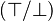$( \btrue / \bfalse )$

Note: In order to simplify and avoid to make a distinction about the predicate sign in the reasoner algorithm, the sub-predicate should be positive.

Afterwards, the hypotheses and the goal are traversed in order to get the list of possible applications of substitutes. Indeed, for each predicate traversed, we retrieve the corresponding substitute previously computed and we ensure that the given predicate won't be rewrite by himself. We store the substitute and the position in the predicate which corresponding to an application of substitutes into the list.

Finally, all applications of substitutes are applied into the sequent, first on the goal and then on the hypotheses. Thus, if the goal is re-written, the re-written goal and the hypotheses needed to achieve it are stored. If the hypotheses are re-written we produce new hypothesis actions.

Note: This improvement corresponds to the level 2 of GenMP.

# Performances

## Configuration

System:

• Intel(R) Core(TM) i7-4770 CPU @ (8* 3.40GHz)
• 24 Go RAM

OS:

• Ubuntu 12.04.3 LTS
• linux kernel 3.5.0-43

Java:

• java-7-openjdk-i386
• VM arguments
• -Xms512m
• -Xmx1024m

Eclipse:

• Indigo (3.7.2)

## Benchmark Projects

The Rodin projects have been taken from the Deploy archives.

## Protocol

A measurement is launched using the plug-in org.eventb.seqprover.core.perf.app. Tests are run from a launched eclipse application.

Launch Configuration must include exactly the following plug-ins:

• org.eventb.core
• org.eventb.core.seqprover
• org.eventb.core.ast
• org.rodinp.core
• org.eventb.core.seqprover.core.per.app

• -application org.eventb.smt.core.perf.app.main <project-dir>

where <project-dir> is the path of the directory where is contained the Rodin projects.

Verify that 1024 Mo are free when tests are ready for launch.

First, it checks that the program argument is a directory and then it imports all Rodin projects from the directory into a workspace. The Auto-prover is applied on all Rodin projects. For each Rodin project the following informations are displayed : "Project: Number of POs discharged/Number of POs". Finally, the proof files are strored into a directory named "Results" at the same level as the directory where is contained the Rodin projects.

## Tests

• Previous version : commit 69e0ae43a1
• New version : commit 2ea1cf6ffe

## Results

The following results are averages of measures on the Auto-prover

Measures
Projects Number Of POs Number Of POs discharged (previous version) Number Of POs discharged (new version)
Doors 108 24 24
ssf 60 11 11
ch915_rev 31 9 9
Celebrity 46 11 11
search_backward 31 8 8
ch916_doors 103 26 26
B2B_mini_pilot_080806 498 272 280
DynamicStableLSR_090624 390 171 171
SimpleLyra 55 33 33
paper_pomc 85 29 30
ch7_conc 248 152 155
ch912_mobile 153 57 57
routing_new 241 86 86
ch915_inv 32 17 18
FloodSet 244 102 103
Rabin-110907 209 70 70
DSAOCSSv003 90 35 35
ch917_train 133 49 49
CDIS 91 40 40
Zer_ess 55 27 27
search_proved 31 10 10
mode_su_12 439 366 382
search 31 10 10
Club-120130 57 21 22
ch2_car 237 143 168
Cowboy-110905 16 9 9
ch915_mini 25 5 5
society_sol 9 0 0
gcd 175 105 105
pattern_poor 88 69 70
ch913_ieee 67 21 21
A2A_081216 276 161 168
ch910_ring 28 8 8
HermanRing-110906 58 24 24
ch911_tree 81 34 36
BoschSwitch 19 9 9
ch4_brp 127 83 86
ProofMethod-130117 14 4 4
ch915_bin 37 12 13
ParkingLot 10 4 4
Galois 1 0 0
ch915_search 17 10 10
ch915_sqrt 17 9 9
ch915_sort 67 25 25
society 7 0 0
BepiColombo_Models_v6.4 155 90 90
proof_obligations 39 18 18
DepSatSpec015Model000 1858 672 1300
ch6_brp 149 100 103
press 60 42 54
SSF_minipilot 24 6 6
ch4_file_1 52 21 21
SignalControl-110527 135 88 93
ResponseCoalitionNormative 178 40 40
ch8_circ_arbiter 153 114 128
pattern_rich 128 112 112
Modes_v2 121 50 50
DiningCryptSpec-100406 24 15 15
Mini_doors 12 4 4
ResponseCoalitionPerturbed 227 42 42
family_sol 9 1 1
Total 8161 3786 4518
Measures
Projects Number Of POs Time (previous version) Time (new version)
DepSatSpec015Model000 1858 2192,036 s (~36 min) 1811,398 s (~30 min)

## Conclusion

We measured that we had an overall 19,3 % increase in number of POs discharged compared to the previous version. A less time of few minutes has been observed for discharge the POs. Only one time measurement has been taken into account because the others are too short. Moreover, this result is not really significiant because even if the new version discharges more sequent than the previous, it decharges earlier than the previous version.